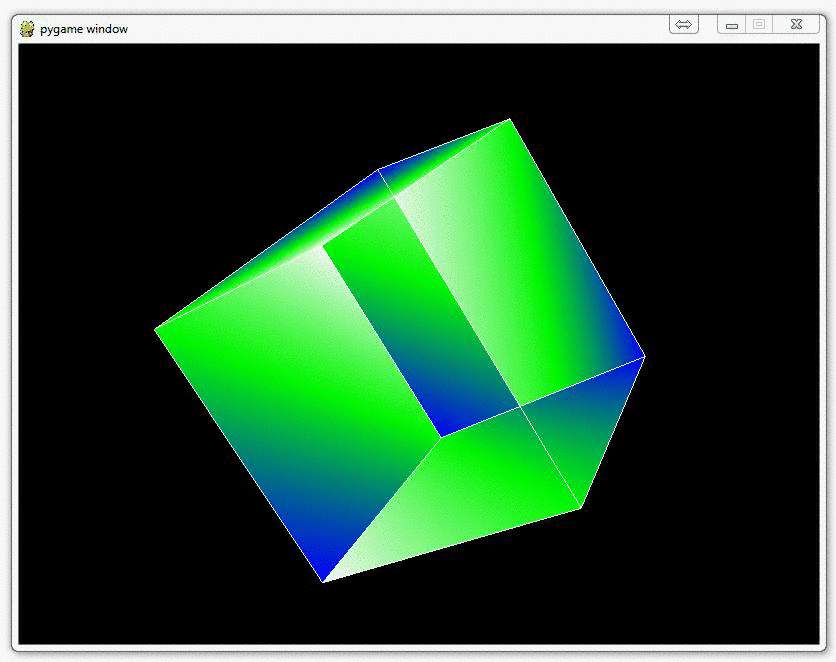## Coloring Surfaces as well as understand some of the basic OpenGL code

In this PyOpenGL tutorial series for OpenGL with Python 3, we're going to discuss how you can color things, specifically surfaces. The way we color surfaces in OpenGL is by first notifying OpenGL that is what we're going to do. After that, we inform OpenGL where this "surface" to be colored is. In our case, each surface is between the connection of four vertices. Other than that, and one new OpenGL function, you're done!

So, first we need some colors to choose from. OpenGL wants you to specify colors in an RGB format, but OpenGL expects it to be between 0 and 1, where 1 is the "strongest."

For example, a nice solid green would be: (0,1,0), which translates to 0 red, 1 green, 0 blue, or no red, full green, no blue.

Now let's go ahead and define a tuple of color tuples:

```colors = (
(1,0,0),
(0,1,0),
(0,0,1),
(0,1,0),
(1,1,1),
(0,1,1),
(1,0,0),
(0,1,0),
(0,0,1),
(1,0,0),
(1,1,1),
(0,1,1),
)
```

We wont use all of these yet, and we'll modify these colors likely later.

Next, we need to add the new coloring code into our Cube() function.

The current Cube() function looks like:

```def Cube():
glBegin(GL_LINES)
for edge in edges:
for vertex in edge:
glVertex3fv(verticies[vertex])
glEnd()
```

Next we need to define surfaces. These surfaces are defined as groups of vertices that make up the surface.

```surfaces = (
(0,1,2,3),
(3,2,7,6),
(6,7,5,4),
(4,5,1,0),
(1,5,7,2),
(4,0,3,6)
)```

Now, we want to add the following to this function:

```    glBegin(GL_QUADS)
for surface in surfaces:
x = 0
for vertex in surface:
x+=1
glColor3fv(colors[x])
glVertex3fv(verticies[vertex])
glEnd()
```

So here you see the typical glBegin, only this time we have (GL_QUADS) as the constant. Then, for each surface (a collection of vertices) in the surfaces tuple, then for each vertex in that list of four vertices, we want to us glColor3fv, which is what will color the object we're creating here, then we use glVertex3fv, just like we had before!

The only other thing here is a crude counter, which adds 1 to the x value, which allows us to create a bit of a multi-colored cube.

Now our Cube() function looks like this:

```def Cube():
for surface in surfaces:
x = 0
for vertex in surface:
x+=1
glColor3fv(colors[x])
glVertex3fv(verticies[vertex])
glEnd()

glBegin(GL_LINES)
for edge in edges:
for vertex in edge:
glVertex3fv(verticies[vertex])
glEnd()
```

Awesome, that's it. Now you have:The next tutorial:• OpenGL with PyOpenGL introduction and creation of Rotating Cube

• Coloring Surfaces as well as understand some of the basic OpenGL code
• Understanding navigation within the 3D environment via OpenGL

• Moving the player automatically towards the cube

• Random Cube Position

• Adding Many Cubes to our Game

• Adding a ground in OpenGL

• Infinite flying cubes

• Optimizing the processing for infinite cubes